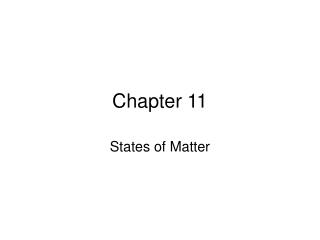DownloadDownload PresentationChapter 11

# Chapter 11

Télécharger la présentation## Chapter 11

- - - - - - - - - - - - - - - - - - - - - - - - - - - E N D - - - - - - - - - - - - - - - - - - - - - - - - - - -
##### Presentation Transcript

1. Chapter 11 States of Matter

2. Physical states

3. Property differences among Physical states • Compressibility: measure of volume change resulting from pressure change. • Thermal Expansion: Measure of volume change resulting from temperature change

4. The KMT of Matter: • Five statements used to explain three states. • Matter is composed of discrete tiny particles. • Particles are in constant motion and possess K.E (energy because of motion). • Particles interact through attractions and repulsions and possess P.E(stored energy).

5. The KMT of Matter: • Particles of opposite charges attract, like charges repel. • Electrostatic force: An attractive force or repulsive force that occurs between charged particles. • The K.E (velocity) increases as temp increases. • Particles in a system transfer energy through elastic (total KE remains constant) collisions.

6. The Solid State: • Physical state characterized by a dominance of P.E (cohesive forces) over K.E (disruptive forces). • Strong cohesive forces hold particles in fixed positions, def S and def V. • Large number of particles in a unit volume, high density.

7. The Solid State: • Very little space in-between particles, small compressibility. • Increase in temp causes K.E to increase , very small thermal expansion.

8. The Liquid state: • Physical state characterized by P.E and K.E of same magnitude. • Definite volume and indefinite shape. • High density: Particles not widely spread. • Small compressibility: Very less empty space. • Small thermal expansion

9. The Gaseous state: • Physical state characterized by a complete dominance of K.E over P.E • Indefinite V and Shape. • Low density: Particles are widely separated and few of them present in a given volume. • Large compressibility: Particles widely separated. • Moderate thermal expansion: Volume increases with increase in temp.

10. A Comparison of Solids, Liquids and Gases • In gases particles are far away from each other compared to solids and liquids. • The distance ratio between particles of s, l, g: 1 to 1.1 to 10

11. Endothermic/Exothermic • Endothermic: System absorbs energy. Ex: melting, sublimation, evaporation. • Exothermic: System releases (exits) energy. Ex: deposition, condensation, freezing.

12. Heat energy and Specific Heat: • The SI unit for heat energy is the joule (pronounced “jool”). • Another unit is the calorie. • 1 Joule of energy is required to raise the temperature of 1 g of water by 1 C. • 1Calorie= 4.184 J. • 1kcal= 4.184 kJ.

13. Problems: • 1)Convert 55.2 kJ into joules, kilocalories and calories. • 2)Convert 11,900 calories into joules, kilocalories and kilojoules.

14. Specific Heat: • The specific heat of a substance is the quantity of heat required to change the temperature of 1 g of that substance by 1oC. • The units of specific heat in joules are: J/g C • Q= mc∆t • Q= heat in J, m= mass in g, c= specific heat in J/g C, ∆t= change in temperature in C

15. Problems • 3)Calculate the specific heat of a solid in J/goC and in cal/ goC if 1638 J raise the temperature of 125 g of the solid from 25.0oC to 52.6oC. • 4)Calculate the number of Joules of heat energy needed to increase the temperature of 50.0 g of Cu from 21.0 C to 80.0 C. c of Cu= 0.382 J/g C.

16. Evaporation of Liquids • Evaporation: Process by which molecules escape from a liquid to a gaseous phase. • Vapor: Gaseous sate of a substance at a temperature and pressure at which substance is normally a liquid or solid. • Equilibrium state: Two opposite processes take place at same rate.

17. Vapor Pressure of liquids • Vapor pressure: Pressure exerted by a vapor above a liquid when liquid and vapor are at equilibrium. • Volatile: Readily evaporates at RT. • Boiling: Conversion from liquid to vapor (evaporation) occurs within the body through bubble formation.

18. Vapor Pressure of liquids • Boiling point: Temperature of a liquid at which the vapor pressure of the liquid becomes equal to the external atmospheric pressure exerted on the liquid. • Normal boiling point: Temperature of liquid at which it boils under a pressure of 760 mm Hg.

19. Intermolecular forces in Liquids • Intermolecular force: Attractive forces between molecules. • Weak forces compared to intra molecular.

20. Within molecules,chem bonds Between molecules

21. Dipole-Dipole interactions • Dipole-Dipole interactions: occurs between polar molecules.

22. Hydrogen bond H is covalently bonded to a highly electronegative element of small size(F, O , N). It is a very strong dipole-dipole interaction. Ex: water.

23. London Forces Weakest type of all intermolecular. Occurs between an atom and a molecule.

24. Ion dipole interactions • Occurs between an ion and a polar molecule

25. Ion-Ion interactions Ionic compounds dissolved in water.